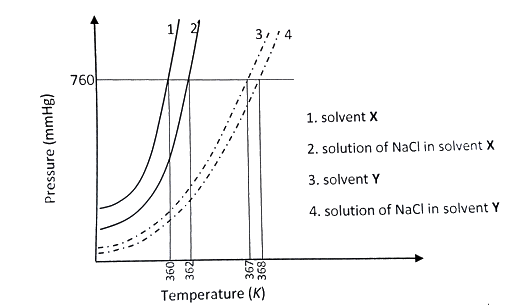The plot given below shows P -T curves (where P is the pressure an | Filoclass 12

Missing549

The plot given below shows curves (where P is the pressure and T is the temperature) for two solvents X and Y and isomolal solutions of NaCl in these solvents. NaCl completely dissociates in both the solvents.On addition of equal number of moles of a non-volatile solute S in equal amount (in kg) of these solvents, the elevation of boiling point of solvent X is three times that of solvent Y. Solute S is known to undergo dimerization in these solvents. If the degree of dimerization is 0.7 in solvent Y, the degree of dimerization in solvent X is ____.549Connecting you to a tutor in 60 seconds.